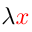Higher-Order Logic Explorer < Previous   Next > Nearby theorems Mirrors  >  Home  >  HOLE Home  >  Th. List  >  ax17 Unicode version

Theorem ax17 205
 Description: Axiom to quantify a variable over a formula in which it does not occur. Axiom C5 in [Megill] p. 444 (p. 11 of the preprint). Also appears as Axiom B6 (p. 75) of system S2 of [Tarski] p. 77 and Axiom C5-1 of [Monk2] p. 113.
Hypothesis
Ref Expression
ax17.1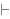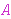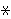Assertion
Ref Expression
ax17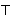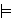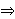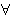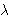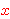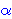Distinct variable groups:,,Proof of Theorem ax17
Dummy variable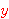is distinct from all other variables.
StepHypRef Expression
1 ax17.1 . 22 wv 58 . . 331, 2ax-17 95 . 2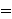41, 3isfree 176 1Colors of variables: type var term Syntax hints:  tv 1hb 3  kc 5kl 6kt 8kbr 9wffMMJ2 11  wffMMJ2t 12tim 111tal 112 This theorem was proved from axioms:  ax-syl 15  ax-jca 17  ax-simpl 20  ax-simpr 21  ax-id 24  ax-trud 26  ax-cb1 29  ax-cb2 30  ax-refl 39  ax-eqmp 42  ax-ded 43  ax-ceq 46  ax-beta 60  ax-distrc 61  ax-leq 62  ax-distrl 63  ax-hbl1 93  ax-17 95  ax-inst 103  ax-eta 165 This theorem depends on definitions:  df-ov 65  df-al 116  df-an 118  df-im 119 This theorem is referenced by: (None)
 Copyright terms: Public domain W3C validator